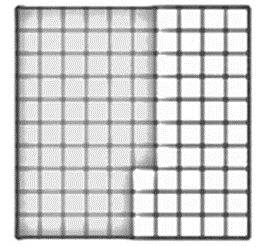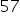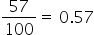Mathematics
Easy

Question

# Choose the decimal for the shaded portion## 0.43430.5757Hint:

## The correct answer is: 0.57

### Total number of square tiles = 100partsShaded part =To convert it into decimal, divide the shaded part by total number of tiles =Thus, 0.57 is the answer.Converting in decimal = 0.57#### With Turito Foundation.#### Get an Expert Advice From Turito.